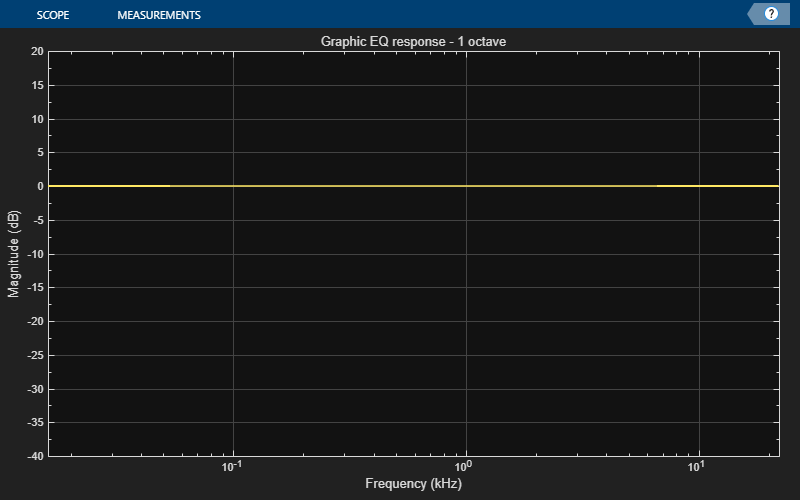# visualize

Visualize magnitude response of graphic equalizer

## Syntax

``visualize(equalizer)``
``visualize(equalizer,NFFT)``
``hvsz = visualize(___)``

## Description

````visualize(equalizer)` plots the magnitude response of the `graphicEQ` object, `equalizer`. The plot is updated automatically when properties of the object change.```

example

````visualize(equalizer,NFFT)` specifies an N-point FFT used to calculate the magnitude response.```
````hvsz = visualize(___)` returns a handle to the visualizer as a `dsp.DynamicFilterVisualizer` object when called with any of the previous syntaxes.```

## Examples

collapse all

Create a default `graphicEQ` System object™ and then call `visualize`.

```equalizer = graphicEQ; visualize(equalizer)```Set the gains of the graphic equalizer to new values. The visualization of the magnitude response updates automatically.

`equalizer.Gains = [-1,1,2,3,3,2,-10,5,5,-10];`## Input Arguments

collapse all

Graphic equalizer whose magnitude response you want to plot.

Number of bins used to calculate the DFT, specified as a positive scalar.

Data Types: `single` | `double`

## Version History

Introduced in R2017b